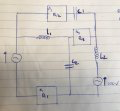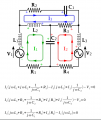# A question about the circuit analysis and system of equations

#### Wouter Lommerse

Joined Aug 27, 2019
2
Hello all,

I'm a student who is busy trying to get a bachelor degree.
Q: write the system of equations to calculate al the currents and voltages?
R1 = 100 ohm
R2 = 150 ohm
R3 = 30 ohm
L1 = 0.5 henry
L2 = 0.2 henry
frequency = 50 hertz#### WBahn

Joined Mar 31, 2012
24,688
Not that this is Homework HELP, not Homework Done For You.

The expectation is that you will show your best attempt, how ever much there is, and that we can then engage in a discussion where we help you get from where you are to where you need to be by nudging you in the direction you need to go or helping you identify errors in your reasoning or work.

#### bertus

Joined Apr 5, 2008
20,048
Hello,

Even at full size, the pictures you posted are to small to read.

Bertus